# The difference in orbits of planets in terms of Newtonian mechanics and Einsteinian mechanics

0
329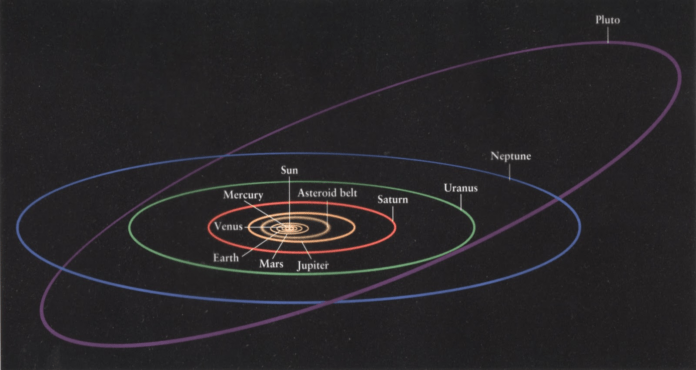In Newtonian mechanics, the orbits of planets are described as elliptical. They result from the interplay between the gravitational attraction between the earth and the central star and the planet’s linear momentum. However, in Einstein’s theory of general relativity, the presence of mass and energy distorts spacetime, leading to a deviation from the purely elliptical orbits described by Newton. The effect is most pronounced in the case of massive bodies, causing the orbits of nearby celestial objects to be affected, resulting in small shifts in their positions over time. This deviation from the predictions of Newtonian mechanics was confirmed by the famous 1919 solar eclipse measurement of the deflection of starlight by the sun, providing strong evidence for the correctness of general relativity. The primary goal of the research paper will be to illustrate the qualitative difference between the two theories of gravity and to contrast the orbits in terms of Newtonian gravity and Einstein’s gravity in terms of their meaning and equation. Because it is a comparison, the reader can identify the difference between the two orbits and their equations, as the method to derive them will be explained in detail and order. The methods would involve solving analytical equations of motion and using numerical solutions to plot and compare the orbits visually.

#### Polar Coordinates

The polar coordinate system is most appropriate where the phenomenon being considered is planar physical systems with bodies moving around a central point. This will be a crucial topic to build our understanding of the position and velocity of an object in orbit, hence making us map it better. In polar coordinates, a point is described using two values: r and. r represents the distance from the origin to the point. It is a positive value and can be any actual number, including zero. Th distance is measured along a ray extending from the origin to the point.represents the angle between the reference direction (usually the positive x-axis) and the ray connecting the origin and the point. The angle is measured in radians and can be any actual number, but it is typically restricted to the range [0,2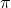) or (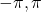] to cover a complete revolution. To illustrate the polar coordinates, we first need to draw a unit circle containing the position vector r in the first quadrant. The angle from the X-axis to the position vector r is the angle?
A unit vector is drawn, originating from and in the same direction as the position vector. Another unit vector is drawn perpendicular to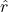like in Figure 1 below.

## Introduction

Gravity was one of the first forces to be recognized and is the foundation of the first laws of motion to be defined in physics.
In our everyday lives, it appears relatively weak compared to its astronomical influence on the bodies of matter throughout the universe1.

According to Newton, gravity was a mysterious force that drew two bodies together. Acting upon that understanding, he developed and altered numerous equations that explained the orbits of planets. Despite doing this, he could never justify the phenomenon, let alone give a reason for its existence. In the years that followed, Einstein would come to explain the reasoning behind gravity by developing the idea of space-time and the general theory of relativity with the help of his famous thought experiments.

This literature review will explain the types of orbits formed by these two ideas of gravity and provide a deeper insight into their graphical differences. In the Newtonian section, we will study the preliminary concepts needed to understand th groundwork of the paper. After that, We will derive an object’s position, velocity, and acceleration to find an equation for its centripetal force, which will be transformed into a graph.

## Derivation of position, velocity, and acceleration

The position of an object in a given orbit is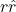with r being the distance of the object from the center of the orbit andrepresenting the direction of r.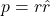Aspoints in the position vector’s direction, they both vary simultaneously. This gives us the equation:When we substitute this in the first equation; we get: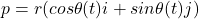#### Velocity

The derivative of the position will be velocity. Hence, the equation:The time derivative of the unit vectoris:Through factorization, we get:Therefore, the velocity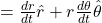#### Acceleration

The derivative of velocity is acceleration.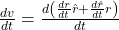Let’s divide it into two parts.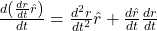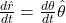Therefore, the first part is: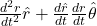2nd part: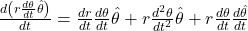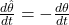Putting the 1st and 2nd parts together, we have:We can simplify it and write: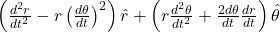## Newton’s law of motion

Newton’s second law of motion states that F = ma When a mass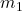is revolving around a larger mass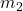, the only force exerted onis going to be a gravitational force2.

Hence, we can write: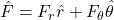The component of force in the direction of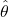is 0 as the gravitational force exerted on m1 is only going to be thecomponent of the total force, which is: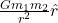## Equations of motion

1. In the radial direction:2. In the angular direction:We will disregard the force in the angular direction and focus on the first equation where we replace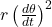with h: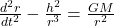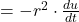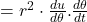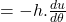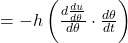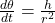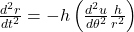Substituting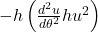in the place of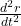gives us: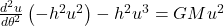Dividing both the sides by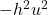gives us: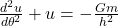Where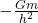is a constant. Because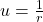, the only possible function that can be used in place of u is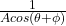,where A affects the orbit size and the orbit angle with respect to its Origin. Depending on the variation of these values, numerous orbits with different tilts and sizes can be created, like the one below.

## Conservation of Angular momentum

There are two types of angular momentum: spin and orbital. We will delve into orbital angular momentum and how the force is conserved.

In the context of orbital motion, such as the motion of planets around the Sun or electrons around an atomic nucleus, orbital angular momentum refers to the rotational motion of the object as it orbits.

Mathematically, the orbital angular momentum (L) of an object can be calculated as the cross product of its position vector (r) and its linear momentum vector (p):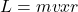To show that the angular momentum is conserved, the time derivative must be 0.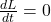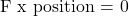Since the direction of the position vector and the force is opposite, their cross-product will be 0, leading to the conservation of angular momentum. In other words, even though the radial and velocity vectors keep changing, the angular momentum of a planet will stay constant in both directions and magnitude.

#### Einstein

The Einstein model of gravity rests upon the premise of general relativity, which was thought of because of the incompatibility of special relativity and Newtonian mechanics. According to Newton’s version of gravity, actions over a distance take effect immediately.

On the other hand, special relativity states that nothing can travel faster than the speed of light. Due to this disparity between theories, Einstein came up with a whole new conception of gravity, the general theory of relativity, which states that the universe is a fabric of space
and time and bends in direct proportion to an object’s mass to display the observed gravitational effect. Ten field equations3 made by Einstein demonstrate this effect and can be used to uncover the modus operandi of the universe itself.

We will use the Schwarzschild solution to the field equations to depict the gravitational field outside a spherically symmetrical mass. This is because the mass has no electric charge, angular momentum, and the universal cosmological constant is zero. The Schwarzschild solution4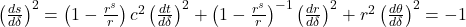Where: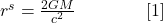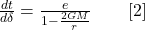Substituting them into the solution above gives us: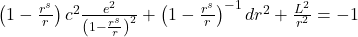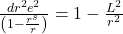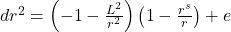On the LHS,can be rewritten as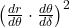, which can be simplified into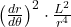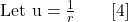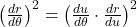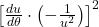By canceling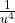with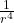we get: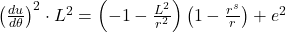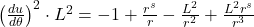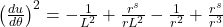By rewriting r as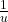, we get: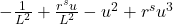By taking the derivative, with respect to theta, on both sides, we get: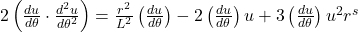Let us take Earth as an example and examine the orbit for the same:Plotting the aforementioned equation in Mathematica will give us this shape:

The above image showcases a spiraling orbit, wherein the body never goes through its starting point again and remains open.

## Discussion

Albert Einstein’s theory of general relativity fundamentally challenged and replaced Isaac Newton’s laws of motion and the law of universal gravitation. Einstein’s theory describes gravitation as a curvature of spacetime caused by mass and energy, whereas Newton saw it as a force between objects. Despite the success of Einstein’s theory in explaining a wide range of phenomena, some gaps in our understanding still require further research.

One such area is the relationship between general relativity and quantum mechanics, which describes the behavior of particles on a tiny scale. There is yet to be a consistent theory that integrates general relativity and quantum mechanics, known as the problem of quantum gravity.

Another area of ongoing research is the nature of dark matter and energy, which comprise most of the universe’s mass-energy content but cannot be directly observed. Einstein’s theory of general relativity does not explain these mysterious substances, and researchers are actively searching for a theoretical framework that can match their existence with our understanding of gravity.

The main difference between the findings of Einstein and Newton in terms of the orbits formed lies in their explanations for the cause of orbital motion. Isaac Newton, in his laws of motion and the law of universal gravitation, explained that objects in orbit around each other are being pulled towards each other by gravitational force. This force results in the objects accelerating toward each other, causing them to move in elliptical orbits. On the other hand, Albert Einstein described gravity as a result of the curvature of spacetime caused by mass and energy. According to Einstein’s theory of general relativity, objects in orbit around a massive body (such as a planet or star) follow the curvature of spacetime rather than being on the receiving end of a force.

It is important to note that Newton’s laws and Einstein’s theory of general relativity have successfully explained a wide range of phenomena and predicted the motion of objects in the universe, including the orbits of planets and stars. However, Einstein’s theory is more accurate and thoroughly explains gravitational phenomena, especially in cases where the gravitational field is strong, such as near black holes.

The general theory of relativity has advanced our understanding of scientific phenomena and predicted several acute effects, such as gravitational redshift and time dilation, which have since been confirmed through experimental and observational evidence.

## Conclusion

This paper illustrates a qualitative difference in the orbits of planets in terms of two concepts. Newtonian mechanics explains the orbits of planets as elliptical, resulting from the gravitational attraction between the earth and the central star. On the other hand, Einstein’s
theory of general relativity describes how mass and energy distort spacetime, causing a deviation from purely elliptical orbits. The paper also acknowledges the ongoing research areas, such as integrating general relativity with quantum mechanics and the nature of dark matter and energy.

Mathematically, the final equation of the orbit, derived from Newtonian mechanics, is:Where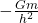is a constant, and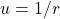By solving this equation analytically, we find out thatOn the other hand, the final equation derived using Einstienian mechanics results in this equation, which can be solved numerically in Mathematica:The orbit produced by the Newtonian equation yields a perfectly elliptical orbit, whereas the orbit made by the Einstienian equation gives us a spiraling orbit, wherein the body never goes through its starting point again and remains open.

Future research into the difference between the orbits of planets according to Newtonian mechanics and Einsteinian mechanics can focus on several key areas. Firstly, further investigation is needed to refine and expand our understanding of the effects of general relativity on planetary orbits in extreme gravitational environments. Integrating general relativity and quantum mechanics is another vital direction, addressing the challenge of quantum gravity and providing a more comprehensive understanding of gravity’s impact on planetary motion. Additionally, studying the dynamics of celestial systems, such as multi-body interactions, through numerical simulations and analytical techniques can yield insights into planetary orbits. Finally, exploring the nature of dark matter and dark energy and their influence on gravity could uncover their effects on planetary motion on cosmological scales. Pursuing these research directions will contribute to advancing our knowledge of gravity and its role in shaping the behavior of planets in the universe.

## References

1. What’s the difference between Newton and Einstein gravity? https://www.skyatnightmagazine.com/space-science/newton-einstein-gravity. []
2. Philosophiæ Naturalis Principia Mathematica, 2023, https://en.wikipedia.org/w/index.php?title=Philosophi%C3%A6_Naturalis_Principia_Mathematica&oldid=1175260336, Page Version ID: 1175260336. []
3. Volume 6: The Berlin Years: Writings, 1914-1917 (English translation supplement) page iv, https://einsteinpapers.press.princeton.edu/vol6-trans/4. []
4. Schwarzschild, 65. On the Gravitational Field of a Point Mass according to the Einsteinian Theory, Harvard University Press, 2013, pp. 451-455. []